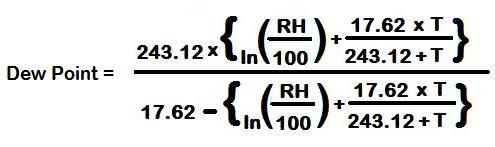CalcuNation

# Dew Point Calculator (Celsius)

## Calculate the dew point in celsius with this Dew Point Calculator.

This calculator uses the Magnus formula to calculate the dew point when the temperature range is between -45 and 60 degrees celsius. Assuming no changes in air pressure.

Temp (Celsius):
Humidity: %
Fill in the temperature and humidity to determine the approximate
dew point.

### What is the Magnus Dew Point Formula?

To calculate the Dew Point with the Magnus Dew Point formula:RH = Relative Humidity (in percent)

T = Temperature (in Celsius)

Example: When the ambient temperature (in celsius) is 50 degrees,
relative humidity is 90%, the approximate dew point is:

Approximate Dew Point = 47.90 Degrees Celsius

### \$100 Promotion

Win \$100 towards teaching supplies! We want to see your websites and blogs.Enter Here

### Calculator Popups

Scientific Calculator
Simple Calculator

### Calculator Ideas

We use your calculator ideas to create new and useful online calculators.
Submit Calculator Idea